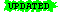The Department of Computer Science & EngineeringCSE 191: DISCRETE STRUCTURES (Fall 2010)Instructor: Prof. William J. Rapaport Times: MWF 12:00 NOON–12:50 P.M. Classroom: 215 NSC

Catalog Description:
Foundational material for further studies in computer science. Topics include logic, proofs, sets, functions, relations, recursion, recurrence relations, mathematical induction, graphs, trees, and some basic counting theory.

CSE 191 is required for computer science and computer engineering majors.Pre- or co-requisites: CSE 113 or CSE 115.

We will begin with a study of logic (propositional and first-order predicate logics), a subject which is at the foundation of mathematics and computer science. Logic can be considered as what AI researchers call a language for knowledge representation and reasoning. As a language, it enables us to talk precisely about anything in mathematics, just as a programming language enables us to talk precisely about computational procedures.

But we need objects to talk about. We will see that we can represent any mathematical or computational object in terms of a single data type: sets (along with their members and the set-membership relation [∈] between them); so, we will study set theory.

Then we'll use the language of logic and the set data-type to investigate relations among objects, including recursive relations, which are at the heart of computer science, as well as investigating functions, which are a special kind of relation (and computable functions are what computer science is all about).

Finally, we'll use logic, set theory, and relations to discuss graphs and trees, yet another very general and useful data type.

Related web pages: